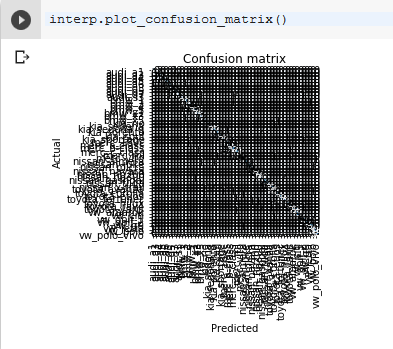# How to expand Confusion Matrix

#1

I am a complete noob. I am trying to identify car types from 57 classes. When I try use the confusion matrix it shows up as a tiny box that is completely unreadable. Is there a way to expand the matrix?0 Likes

(Daniel) #2

You can try the plt.figure command just above your plotting command

``````plt.figure(figsize=(10,15))
interp.plot_confusion_matrix()``````

You can change the numbers to whatever you want.
Another thing that could be helpful is that if you reset the notebook and skip the line %matplotlib inline.
you can then instead use %matplotlib notebook where you can zoom and pan in the plots. But this feature is a bit buggy and need sometimes to be added just above the plot command in every cell where you make a new plot.

0 Likes

#3

Hi
I tried adding that line but the only difference it made was to add a size above the matrix…
<Figure size 1440x1800 with 0 Axes>

0 Likes

#4

I changed the inline to … %matplotlib notebook and got this error:
Warning: Cannot change to a different GUI toolkit: notebook. Using tk instead.

0 Likes

(Daniel) #5

Hello.
I have tried this now and it seems like you need to pass in the figsize into the plot function.

``interp.plot_confusion_matrix(figsize=(10,10))``

I don’t understand why the matplotlib notebook command does not work for you.There are others that had the same problem, I think you need to google around a little.

0 Likes

#6

Thanks @dankraf. This solved my problem.

0 Likes